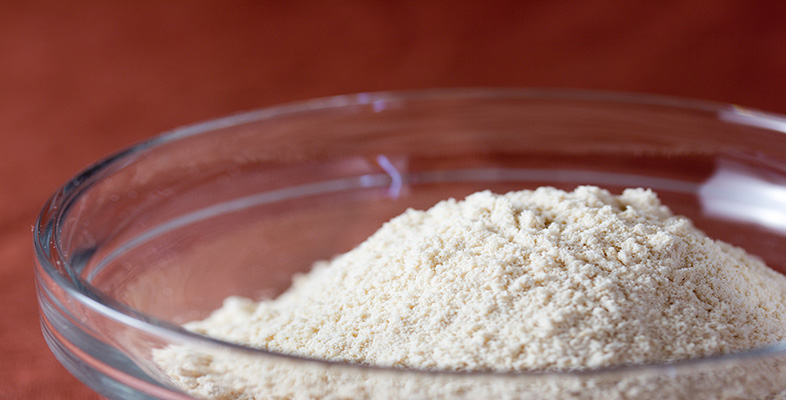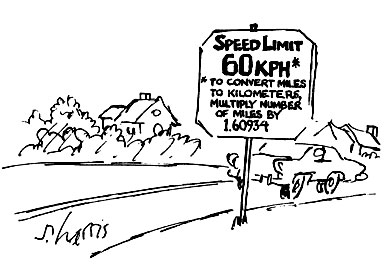Rounding and estimation

Start this free course now. Just create an account and sign in. Enrol and complete the course for a free statement of participation or digital badge if available.

Free course

# 1.4 Rounding decimalsUsing a calculator often gives a long string of digits. For example, 1 ÷ 3 might give .333333333. But very often, for practical purposes, this level of accuracy is too precise to be useful. You try measuring .33333333 metres!

Decimal numbers can be rounded just like whole numbers. For example, 0.33333333 is nearer to 0.3 than to 0.4 and rounding 0.33333333 m to 0.3 m makes it easy to measure. Here the number has been rounded so that it contains one decimal digit; it has been rounded to one decimal place. (We often write ‘d.p.’ for ‘decimal place’.)

Often it is more sensible to measure to the nearest centimetre (0.01 m), in which case 0.3333333 m rounds to 0.33 m. Here the number contains two decimal digits; it has been rounded to two decimal places.

The rule for rounding applies just as well to decimal places as hundreds.

The number of decimal places given, however, indicates the accuracy. So 0.30 indicates accuracy to the nearest 0.01 (two decimal places) whereas 0.3 indicates accuracy to the nearest 0.1 (one decimal place).

The general rule for rounding to a number of decimal places is to look at the digit one place to the right of the number of digits you want to round to, and round up or down depending on whether this digit is ‘5 or more’ or ‘4 or less’.

## Example 2

• (a) In a diary, the following conversion statements were given. Round all the numbers to one decimal place (1 d.p.) in order to give a handy measure to remember when travelling abroad:

• converting miles to kilometres multiply by 1.6093;

• converting feet to metres multiply by 0.3048.

• (b) Round these values so that they are correct to two decimal places (2 d.p.).

• (a) The answer must contain one decimal digit, so look at the second decimal digit and round up or down accordingly.

• miles to kilometres: the second decimal digit is 0 so round down, thus the conversion factor is 1.6 (to 1 d.p.).

• feet to metres: the second decimal digit is 0 so round down, thus the conversion factor is 0.3 (to 1 d. p.).

• (b) To round to 2 decimal places look at the third decimal digit:

• miles to kilometres: the third decimal digit is 9, so round up. Thus the conversion factor is 1.61 (2 d.p.).

• feet to metres: the third decimal digit is 4 so round down. Thus to convert feet to metres multiply by 0.30 (2 d.p.).

Note the use of a zero in the second decimal place. This is necessary to indicate that the value has been rounded to two decimal places.

MU120_4M2